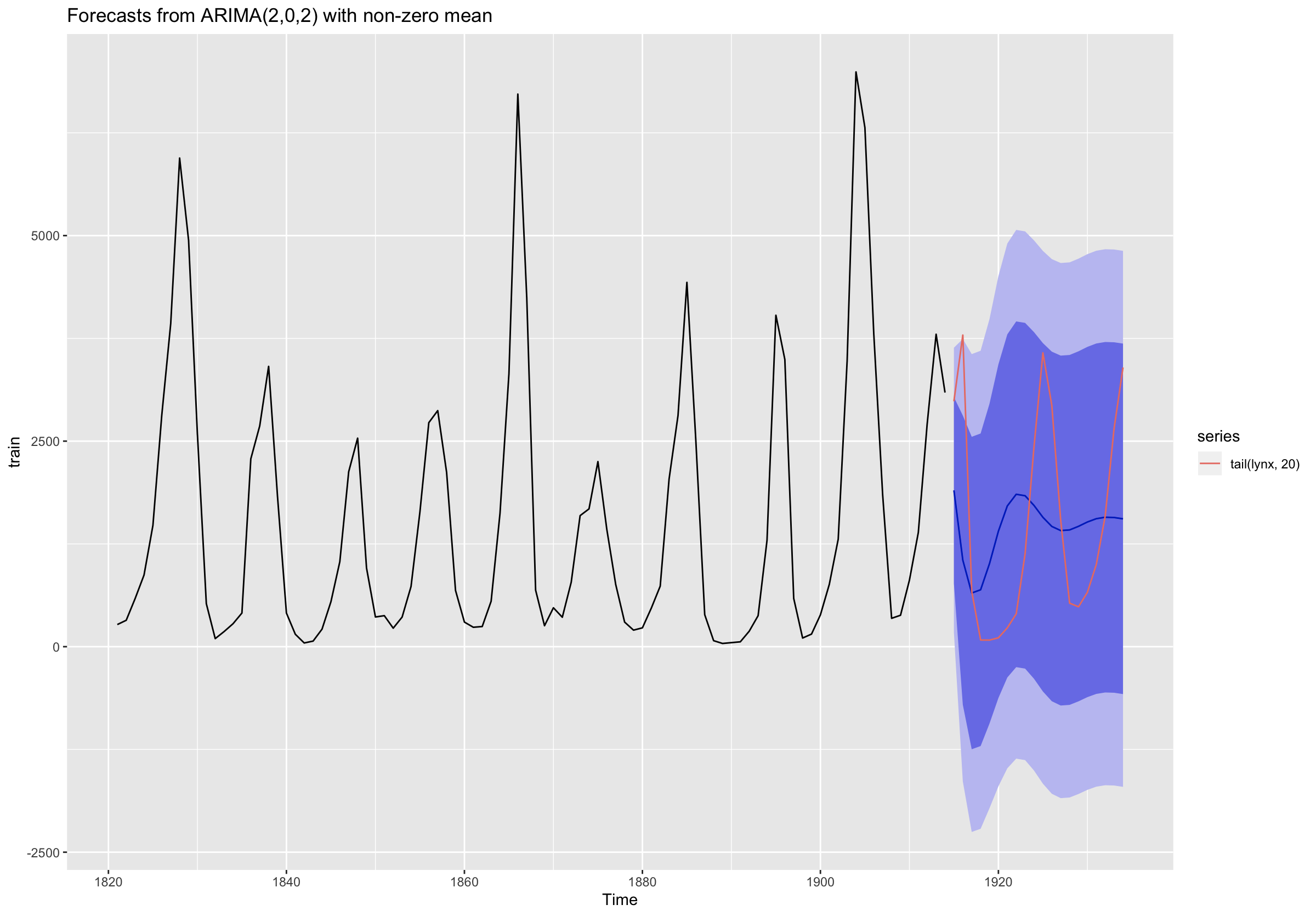Cyclicality in time series

The high amount of cyclicality in the lynx time series makes it very difficult to model with ets and auto.arima.

library(forecast)
library(fpp2)

data(lynx)

train <- subset(lynx, end = length(lynx) - 20) # hold-out last 20 years

ets_mod <- ets(train)
arima_mod <- auto.arima(train)

fc_ets <- forecast(ets_mod, h=20)
fc_arima <- forecast(arima_mod, h=20)
fc_naive <- naive(train, h=20)

accuracy(fc_ets, lynx)
accuracy(fc_arima, lynx) # winner
accuracy(fc_naive, lynx)

autoplot(fc_arima) + autolayer(tail(lynx,20)) # poor fitQuestions:

1. Are there other methods that I should try in the forecast package?

2. How do I change the legend in autoplot() and autolayer() to show "ARIMA Forecast" and "Actual"?